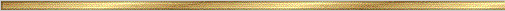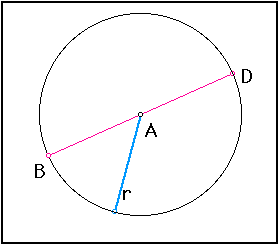# Circumference and Pi Conjecture### Explanation:

The circumference of a circle is the length around the edge of the circle. The diameter is a chord that passes through the center of the circle. The radius is a segment from the center to any point on the circle. You are able to find the circumference once you have the measure of the diameter or the radius.

We see that to find the circumference of a circle we need to use the number "pi". One exploratory way to find pi would be to measure the circumference of circles and divide by their diameters, respectively. You will find that pi is approximately equal to 3.14, or 22/7. Click here to further explore pi.

### The precise statement of the conjecture is:

Conjecture (Circumference ): If C is the circumference and D is the diameter of a circle, then there is a number p such that C=pD. Since D=2r, where r is the radius, the C=2pr.